# Geometric Words You Should Know

Many students work with angles in math class but have no clue how to name those angles. As a math tutor, I face many students who are totally lost in terms of angles. What is an angle? Two rays that share the same endpoint form an angle.

The symbol for angle is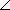. How do we name angles in geometry?

We name angles in three different ways:

(1) We can name angles by using THREE capital letters like:ABC orDEF. The middle letter is called the VERTEX of the angle. The above angles are read "angle ABC" and "angle DEF." This leads us to the second way we can name angles.

(2) We can name angles by using the vertex. For example,ABC, can also be called angle B; the same applies toDEF (we can call angle DEF angle E). Of course, if there's more than one angle sharing the same vertex this would be confusing!

(3) We can also name an angle by placing any number or symbol at the vertex in the INTERIOR of the angle. So, angles can also be called angle 1 or angle 2 or angle 4, etc.

Sample: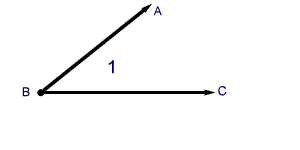The above angle can read three ways:

(1) angle ABC
(2) angle B
(3) angle 1

Sides AB and AC of the given angle are actually rays. Why rays? Because they extend out to infinity in one direction. When two rays meet at a vertex, they form an angle.

In geometry, you will come across postulates and theorems needed for geometric proofs.

A theorem is a statement that can be proved to be true.

A postulate is a statement that is ASSUMED to be true WITHOUT proof. All this becomes part of your new geometry vacabulary. I will go into detail about postulates and theorems in future lessons titled GEOMETRIC PROOFS.

Line Segments: The line segment of a line is the span between two endpoints. Remember, a line itself is infinite, and a line segment is a finite portion of that. Realistically, anything you draw on paper is a line segment because it cannot go on forever!

The degree measure of an angle is the amount of rotation from one side of the angle to other side.

What is a rotation?

A rotation is a movement of an object in a circular motion. Think about the Earth going around the Sun, a journey that takes one year to complete. An angle is a measure of how far around something has gone.

Perpendicular Line: Perpendicular lines are lines that meet or cross to form a right angle. A right angle equal 90 degrees.

A perpendicular symbol is simply an upside down capital letter T. It looks like this: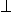Adjacent angles: Two angles are adjacent if they have the SAME VERTEX, share ONE SIDE and do not overlap.

Sample: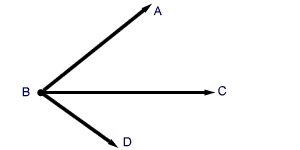The above symbol matches our definition of adjacent angles.

A few more definitions and that's it for Geometry 1.3:

Congruent Segment: When two line segments share the same length, they are said to be CONGRUENT or equal.

Say line segment AB = 10 inches and line segment CD = 10 inches, then line segment AB is congruent or equal to line segment CD.

The symbol for CONGRUENCE is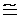and so, the above can be written this way:

We write: ABCD. This means "line segment AB is congruent to line segment CD."

Midpoint of Line Segment: The midpoint of a line segment divides the segment into TWO segments of equal length.

Sample:

Line segment AB is divided by the midpoint M. The distance from A to M is 20 feet and the distance from BM is 20 feet. Then line segment AM = line segment BM.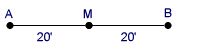Any line or segment that passes through the midpoint of a line segment, bisects (cuts) the line segment.

Lesson provided by Mr. Feliz